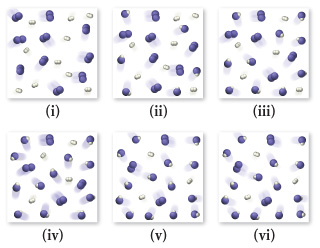# Problem: H2 and I2 are combined in a flask and allowed to react according to the following reaction: H2(g) + I2(g) ⇌ 2 HI(g)Examine the figures (sequential in time) and answer the following questions.Would the final figure (vi) have different amounts of reactants and products in the presence of a catalyst?

⚠️Our tutors found the solution shown to be helpful for the problem you're searching for. We don't have the exact solution yet.

###### Problem Details

H2 and I2 are combined in a flask and allowed to react according to the following reaction: H2(g) + I2(g) ⇌ 2 HI(g)
Examine the figures (sequential in time) and answer the following questions.Would the final figure (vi) have different amounts of reactants and products in the presence of a catalyst?